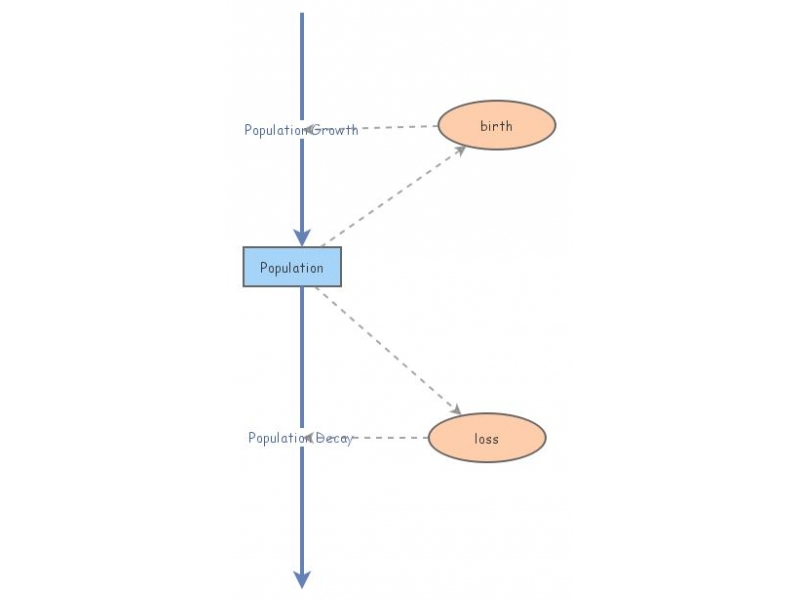Non-dimensionalized Logistic GrowthThis (simplest!) model demonstrates logistic growth.The original differential equation looks like

y'(t) = b y(t) (1 - y(t)/K)

where K is the carrying capacity of the quantity y.

But if we divide each side of the equation by K, we obtain

d(y/K)/dt = b (y/K) (1-y/K)

Defining a new variable w, the population relative to its carrying capacity, we obtain

dw/dt = b w (1 - w)

Finally we divide both sides by b, to write

dw/d(bt) = w (1 - w)

So if we work in dimensionless time units of bt, we have

w' = w (1 - w)

where the derivative is with respect to the variable bt=τ. .
τ=τ
This
This equation, as simple as possible, contains all the dynamics (all the ways the population can behave), while masking the "trivialities"; but it kind of hides the physical aspects of the problem. So it's easy to study, but harder to interpret: alas, you can't have it all!:)

τ=1 when t=1b: so if b=.5/year, then τ=1 when t=2.

So the larger b (the greater the birthrate), the shorter the real time t to give τ=1.
τ=τ=

τ=